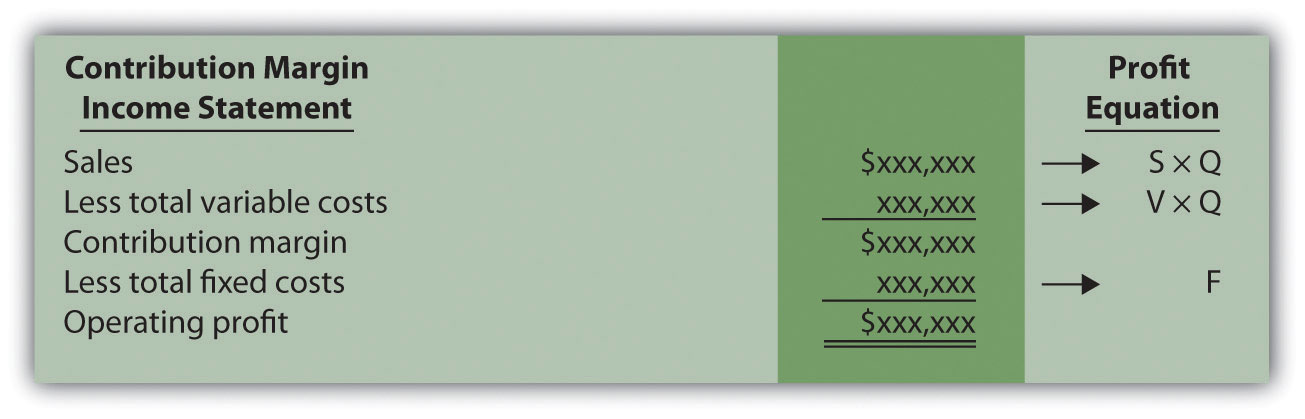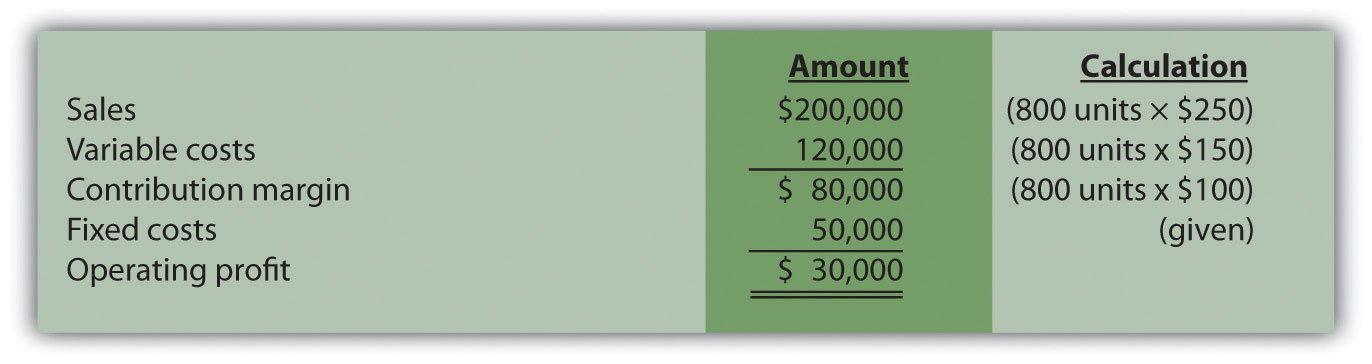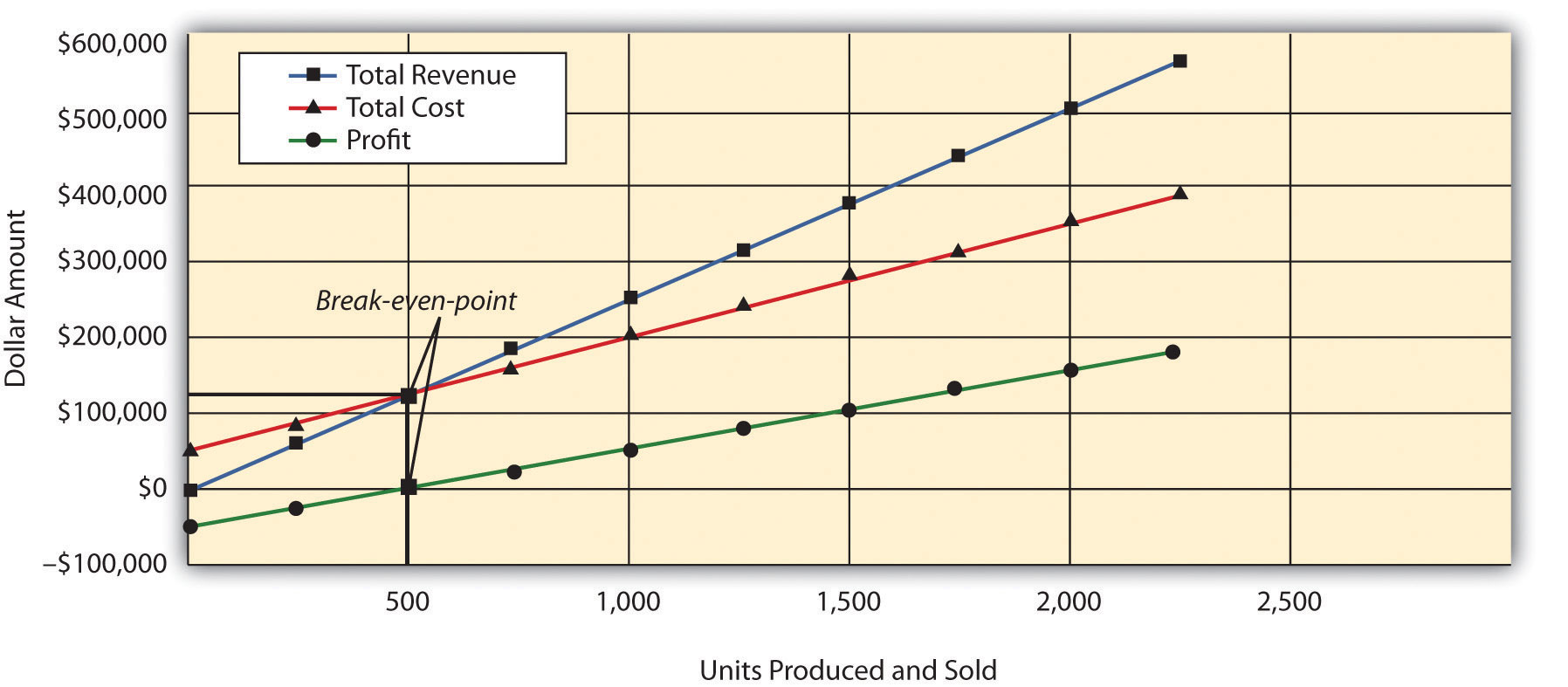## 6.1 Cost-Volume-Profit Analysis for Single-Product Companies

### Learning Objective

1. Perform cost-volume-profit analysis for single-product companies.

Question: The profit equationProfit equals total revenues minus total variable costs minus total fixed costs. shows that profit equals total revenues minus total variable costs and total fixed costs. This profit equation is used extensively in cost-volume-profit (CVP) analysis, and the information in the profit equation is typically presented in the form of a contribution margin income statement (first introduced in Chapter 5 "How Do Organizations Identify Cost Behavior Patterns?"). What is the relationship between the profit equation and the contribution margin income statement?

Answer: Recall that the contribution margin income statement starts with sales, deducts variable costs to determine the contribution margin, and deducts fixed costs to arrive at profit. We use the term “variable cost” because it describes a cost that varies in total with changes in volume of activity. We use the term “fixed cost” because it describes a cost that is fixed (does not change) in total with changes in volume of activity.

To allow for a mathematical approach to performing CVP analysis, the contribution margin income statement is converted to an equation using the following variables:

### Key Equation

S = Selling price per unit V = Variable cost per unit F = Total fixed costs Q = Quantity of units produced and sold

Thus

Figure 6.1 "Comparison of Contribution Margin Income Statement with Profit Equation" clarifies the link between the contribution margin income statement presented in Chapter 5 "How Do Organizations Identify Cost Behavior Patterns?" and the profit equation stated previously. Study this figure carefully because you will encounter these concepts throughout the chapter.

Figure 6.1 Comparison of Contribution Margin Income Statement with Profit EquationRecall that when identifying cost behavior patterns, we assume that management is using the cost information to make short-term decisions. Variable and fixed cost concepts are useful for short-term decision making. The short-term period varies, depending on a company’s current production capacity and the time required to change capacity. In the long term, all cost behavior patterns are likely to change.

## Break-Even and Target Profit

Question: Companies such as Snowboard Company often want to know the sales required to break even, which is called the break-even point. What is meant by the term break-even point?

Answer: The break-even point can be described either in units or in sales dollars. The break-even point in unitsThe number of units that must be sold to achieve zero profit. is the number of units that must be sold to achieve zero profit. The break-even point in sales dollarsThe total sales measured in dollars required to achieve zero profit. is the total sales measured in dollars required to achieve zero profit. If a company sells products or services easily measured in units (e.g., cars, computers, or mountain bikes), then the formula for break-even point in units is used. If a company sells products or services not easily measured in units (e.g., restaurants, law firms, or electricians), then the formula for break-even point in sales dollars is used.

## Break-Even Point in Units

Question: How is the break-even point in units calculated, and what is the break-even point for Snowboard Company?

Answer: The break-even point in units is found by setting profit to zero using the profit equation. Once profit is set to zero, fill in the appropriate information for selling price per unit (S), variable cost per unit (V), and total fixed costs (F), and solve for the quantity of units produced and sold (Q).

## Profit Equation

Question: Let’s formalize this discussion by using the profit equation. How is the profit equation used to find a target profit amount in units?

Answer: Finding the target profit in units is similar to finding the break-even point in units except that profit is no longer set to zero. Instead, set the profit to the target profit the company would like to achieve. Then fill in the information for selling price per unit (S), variable cost per unit (V), and total fixed costs (F), and solve for the quantity of units produced and sold (Q):

Thus Snowboard Company must produce and sell 800 snowboards to achieve $30,000 in profit. This answer is confirmed in the following contribution margin income statement:## Shortcut Formula Question: Although using the profit equation to solve for the break-even point or target profit in units tends to be the easiest approach, we can also use a shortcut formula derived from this equation. What is the shortcut formula, and how is it used to find the target profit in units for Snowboard Company? Answer: The shortcut formula is as follows: ### Key Equation Q = (F + Target Profit) ÷ (S − V) If you want to find the break-even point in units, set “Target Profit” in the equation to zero. If you want to find a target profit in units, set “Target Profit” in the equation to the appropriate amount. To confirm that this works, use the formula for Snowboard Company by finding the number of units produced and sold to achieve a target profit of$30,000:

The result is the same as when we used the profit equation.

## Break-Even Point in Sales Dollars

Question: Finding the break-even point in units works well for companies that have products easily measured in units, such as snowboard or bike manufacturers, but not so well for companies that have a variety of products not easily measured in units, such as law firms and restaurants. How do companies find the break-even point if they cannot easily measure sales in units?

Answer: For these types of companies, the break-even point is measured in sales dollars. That is, we determine the total revenue (total sales dollars) required to achieve zero profit for companies that cannot easily measure sales in units.

Finding the break-even point in sales dollars requires the introduction of two new terms: contribution margin per unit and contribution margin ratio.

## Contribution Margin per Unit

The contribution margin per unitThe amount each unit sold contributes to (1) covering fixed costs and (2) increasing profit. is the amount each unit sold contributes to (1) covering fixed costs and (2) increasing profit. We calculate it by subtracting variable costs per unit (V) from the selling price per unit (S).

### Key Equation

Contribution margin per unit = S − V

For Snowboard Company the contribution margin is $100: Thus each unit sold contributes$100 to covering fixed costs and increasing profit.

## Contribution Margin Ratio

The contribution margin ratioThe contribution margin as a percentage of sales; it measures the amount each sales dollar contributes to (1) covering fixed costs and (2) increasing profit; also called contribution margin percent. (often called contribution margin percent) is the contribution margin as a percentage of sales. It measures the amount each sales dollar contributes to (1) covering fixed costs and (2) increasing profit. The contribution margin ratio is the contribution margin per unit divided by the selling price per unit. (Note that the contribution margin ratio can also be calculated using the total contribution margin and total sales; the result is the same.)

### Key Equation

Contribution margin ratio = (S − V) ÷ S

For Snowboard Company the contribution margin ratio is 40 percent:

## CVP Graph

Question: The relationship of costs, volume, and profit can be displayed in the form of a graph. What does this graph look like for Snowboard Company, and how does it help management evaluate financial information related to the production of snowboards?

Answer: Figure 6.2 "CVP Graph for Snowboard Company" shows in graph form the relationship between cost, volume, and profit for Snowboard Company. The vertical axis represents dollar amounts for revenues, costs, and profits. The horizontal axis represents the volume of activity for a period, measured as units produced and sold for Snowboard.

There are three lines in the graph:

• Total revenue
• Total cost
• Profit

The total revenue line shows total revenue based on the number of units produced and sold. For example, if Snowboard produces and sells one unit, total revenue is $250 (= 1 ×$250). If it produces and sells 2,000 units, total revenue is $500,000 (= 2,000 ×$250).

The total cost line shows total cost based on the number of units produced and sold. For example, if Snowboard produces and sells one unit, total cost is $50,150 [=$50,000 + (1 × $150)]. If it produces and sells 2,000 units, total cost is$350,000 [= $50,000 + (2,000 ×$150)].

The profit line shows profit or loss based on the number of units produced and sold. It is simply the difference between the total revenue and total cost lines. For example, if Snowboard produces and sells 2,000 units, the profit is $150,000 (=$500,000 − $350,000). If no units are sold, a loss is incurred equal to total fixed costs of$50,000.

Figure 6.2 CVP Graph for Snowboard Company## Margin of Safety

Question: Managers often like to know how close projected sales are to the break-even point. How is this information calculated and used by management?

Answer: The excess of projected sales over the break-even point is called the margin of safetyThe excess of expected sales over the break-even point, measured in units and in sales dollars.. The margin of safety represents the amount by which sales can fall before the company incurs a loss.

### Key Equation

Margin of safety (in units) = Projected sales (in units) − Break-even sales (in units)

Assume Snowboard Company expects to sell 700 snowboards and that its break-even point is 500 units; the margin of safety is 200 units. The calculation is

Thus sales can drop by 200 units per month before the company begins to incur a loss.

The margin of safety can also be stated in sales dollars.

### Key Equation

Margin of safety (in sales $) = Projected sales (in sales$) − Break-even sales (in sales $) For Snowboard the margin of safety in sales dollars is$50,000:

Thus sales revenue can drop by $50,000 per month before the company begins to incur a loss. ### Key Takeaway • Cost-volume-profit analysis involves finding the break-even and target profit point in units and in sales dollars. The key formulas for an organization with a single product are summarized in the following list. Set the target profit to$0 for break-even calculations, or to the appropriate profit dollar amount for target profit calculations. The margin of safety formula is also shown:

• Break-even or target profit point measured in units:

(The denominator is also called “contribution margin per unit.”)

• Break-even or target profit point measured in sales dollars:

• Margin of safety in units or sales dollars:

Projected sales − Break-even sales

### Review Problem 6.1

Star Symphony would like to perform for a neighboring city. Fixed costs for the performance total $5,000. Tickets will sell for$15 per person, and an outside organization responsible for processing ticket orders charges the symphony a fee of $2 per ticket. Star Symphony expects to sell 500 tickets. 1. How many tickets must Star Symphony sell to break even? 2. How many tickets must the symphony sell to earn a profit of$7,000?
3. How much must Star Symphony have in sales dollars to break even?
4. How much must Star Symphony have in sales dollars to earn a profit of $7,000? 5. What is the symphony’s margin of safety in units and in sales dollars? Solution to Review Problem 6.1 Note: All solutions are rounded. 1. The symphony must sell 385 tickets to break even: 2. The symphony must sell 923 tickets to make a profit of$7,000:

3. The symphony must make $5,769 in sales to break even: 4. The symphony must make$13,846 in sales to earn a profit of $7,000: 5. The symphony’s margin of safety is 115 units or$1,725 in sales: